# User:Twine

Week 6, Lecture 3

Constant Coefficient Homogeneous High Order ODEs

Ex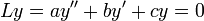$Ly = a y'' + b y' + c y = 0$,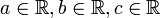$a \in \mathbb{R}, b \in \mathbb{R}, c \in \mathbb{R}$

Or generally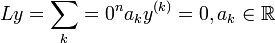$Ly = \sum_k=0^n a_k y^{(k)} = 0, a_k \in \mathbb{R}$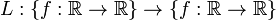$L:\{f: \mathbb{R} \rightarrow \mathbb{R}\} \rightarrow \{f: \mathbb{R} \rightarrow \mathbb{R}\}$ is a linear transformation ("linear operator").

What do we expect from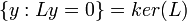$\{y: Ly = 0\} = ker(L)$? We expect an n-dimensional vector space.

Take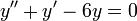$y''+y'-6y = 0$, guess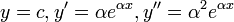$y = c, y' = \alpha e^{\alpha x}, y'' = \alpha^2e^{\alpha x}$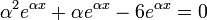$\alpha^2 e^{\alpha x} + \alpha e^{\alpha x} - 6 e^{\alpha x} = 0$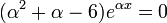$(\alpha^2 +\alpha - 6) e^{\alpha x} = 0$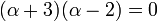$(\alpha +3)(\alpha - 2) = 0$

So we have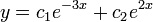$y = c_1e^{-3x} +c_2 e^{2x}$ as the general solution.

Say we have complex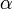$\alpha$. Then what?